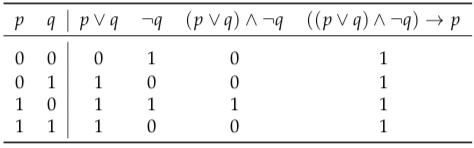# 2.1.10: 2.1.J Classifying propositions

Certain types of proposition will play a special role in our further work with logic. In particular, we define tautologies, contradictions, and contingencies as follows:

Definition 2.4.

A compound proposition is said to be a tautology if and only if it is true for all possible combinations of truth values of the propositional variables which it contains. A compound proposition is said to be a contradiction if and only if it is false for all possible combinations of truth values of the propositional variables which it contains. A compound proposition is said to be a contingency if and only if it is neither a tautology nor a contradiction.

For example, the proposition ((p q) ∧ ¬q) → p is a tautology. This can be checked 2with a truth table:The fact that all entries in the last column are true tells us that this expression is a tautology. Note that for any compound proposition P, P is a tautology if and only if ¬Pis a contradiction. (Here and in the future, I use uppercase letters to represent compound propositions. P stands for any formula made up of simple propositions, propositional variables, and logical operators.)

Logical equivalence can be defined in terms of tautology:

Definition2.5.

Two compound propositions, P and Q, are said to be logically equivalent if and only if the proposition P Q is a tautology.

The assertion that P is logically equivalent to Q will be expressed symbolically as “P Q”. For example, (p q) ≡ (¬p q), and p q ≡ (p q) ∧ ¬(p q).

What if P Q and P is false? From a false premise we can derive any conclusion (check the truth table of →). So if k stands for “I’m the King of the Netherlands”, then k Q is true for any compound proposition Q. You can substitute anything for Q, and the implication k Q will hold. For example, it a logically valid deduction that: If I’m the King of the Netherlands, then unicorns exist. Taking this further, from a contradiction we can derive any conclusion. This is called the Principle of Explosion.

Exercises

1. Give the three truth tables that define the logical operators ∧, ∨, and ¬.

2. Some of the following compound propositions are tautologies, some are contradictions, and some are neither (i.e.,, so are contingencies). In each case, use a truth table to decide to which of these categories the proposition belongs:

a) (p ∧ (p q)) → q b) ((p q) ∧ (q r)) → (p r)

c) p ∧ ¬p d) (p q) → (p q)
e) p ∨ ¬p f) (p q) → (p q)

3. Use truth tables to show that each of the following propositions is logically equivalent to p q.

a) (p q) ∧ (q p) b) ¬p ↔ ¬q
c) (p q) ∧ (¬p → ¬q) d) ¬(p q)

4. Is → an associative operation? This is, is (p q) → r logically equivalent to p → (q r)? Is↔ associative?

1. Let p represent the proposition “You leave” and let q represent the proposition “I leave”. Ex- press the following sentences as compound propositions using p and q, and show that they are logically equivalent:

a) Either you leave or I do. (Or both!) b) If you don’t leave, I will.

2. Suppose that m represents the proposition “The Earth moves”, c represents “The Earth is the centre of the universe”, and g represents “Galileo was falsely accused”. Translate each of the following compound propositions into English:

a) ¬g c b) m → ¬c

c) m ↔ ¬c d) (m g) ∧ (c → ¬g)

7. Give the converse and the contrapositive of each of the following English sentences:

a) If you are good, Sinterklaas brings you toys.

b) If the package weighs more than one kilo, then you need extra postage.
c) If I have a choice, I don’t eat courgette.

8. In an ordinary deck of fifty-two playing cards, for how many cards is it truea) that “This card is a ten and this card is a heart”?

b) that “This card is a ten or this card is a heart”?
c) that “If this card is a ten, then this card is a heart”?

d) that “This card is a ten if and only if this card is a heart”?

9.Define a logical operator ↓ so that p q is logically equivalent to ¬(p q). (This operator is usually referred to as ‘nor’, short for ‘not or’.) Show that each of the propositions ¬p, p q,p q, p q, p q, and p q can be rewritten as a logically equivalent proposition that uses↓ as its only operator.

10. For our proof that {¬, ∨} is functionally complete, we need to show that all formulas in pro- positional logic can be expressed in an equivalent form using only {¬, ∧, ∨, →, ↔}.

a) How many unique truth tables exist for formulas containing two atoms?

b) Create a function for each of the possible truth tables that uses only the 5 operators listed

above.

c) Give an (informal) argument why this means all formulas in propositional logic can be

expressed using only these five operators.